# One-way ANOVA in SPSS Statistics

## Introduction

The one-way analysis of variance (ANOVA) is used to determine whether there are any statistically significant differences between the means of two or more independent (unrelated) groups (although you tend to only see it used when there are a minimum of three, rather than two groups). For example, you could use a one-way ANOVA to understand whether exam performance differed based on test anxiety levels amongst students, dividing students into three independent groups (e.g., low, medium and high-stressed students). Also, it is important to realize that the one-way ANOVA is an omnibus test statistic and cannot tell you which specific groups were statistically significantly different from each other; it only tells you that at least two groups were different. Since you may have three, four, five or more groups in your study design, determining which of these groups differ from each other is important. You can do this using a post hoc test (N.B., we discuss post hoc tests later in this guide).

Note: If your study design not only involves one dependent variable and one independent variable, but also a third variable (known as a "covariate") that you want to "statistically control", you may need to perform an ANCOVA (analysis of covariance), which can be thought of as an extension of the one-way ANOVA. To learn more, see our SPSS Statistics guide on ANCOVA. Alternatively, if your dependent variable is the time until an event happens, you might need to run a Kaplan-Meier analysis.

This "quick start" guide shows you how to carry out a one-way ANOVA using SPSS Statistics, as well as interpret and report the results from this test. Since the one-way ANOVA is often followed up with a post hoc test, we also show you how to carry out a post hoc test using SPSS Statistics. However, before we introduce you to this procedure, you need to understand the different assumptions that your data must meet in order for a one-way ANOVA to give you a valid result. We discuss these assumptions next.

## Assumptions

When you choose to analyse your data using a one-way ANOVA, part of the process involves checking to make sure that the data you want to analyse can actually be analysed using a one-way ANOVA. You need to do this because it is only appropriate to use a one-way ANOVA if your data "passes" six assumptions that are required for a one-way ANOVA to give you a valid result. In practice, checking for these six assumptions just adds a little bit more time to your analysis, requiring you to click a few more buttons in SPSS Statistics when performing your analysis, as well as think a little bit more about your data, but it is not a difficult task.

Before we introduce you to these six assumptions, do not be surprised if, when analysing your own data using SPSS Statistics, one or more of these assumptions is violated (i.e., is not met). This is not uncommon when working with real-world data rather than textbook examples, which often only show you how to carry out a one-way ANOVA when everything goes well! However, don’t worry. Even when your data fails certain assumptions, there is often a solution to overcome this. First, let’s take a look at these six assumptions:

• Assumption #1: Your dependent variable should be measured at the interval or ratio level (i.e., they are continuous). Examples of variables that meet this criterion include revision time (measured in hours), intelligence (measured using IQ score), exam performance (measured from 0 to 100), weight (measured in kg), and so forth. You can learn more about interval and ratio variables in our article: Types of Variable.
• Assumption #2: Your independent variable should consist of two or more categorical, independent groups. Typically, a one-way ANOVA is used when you have three or more categorical, independent groups, but it can be used for just two groups (but an independent-samples t-test is more commonly used for two groups). Example independent variables that meet this criterion include ethnicity (e.g., 3 groups: Caucasian, African American and Hispanic), physical activity level (e.g., 4 groups: sedentary, low, moderate and high), profession (e.g., 5 groups: surgeon, doctor, nurse, dentist, therapist), and so forth.
• Assumption #3: You should have independence of observations, which means that there is no relationship between the observations in each group or between the groups themselves. For example, there must be different participants in each group with no participant being in more than one group. This is more of a study design issue than something you can test for, but it is an important assumption of the one-way ANOVA. If your study fails this assumption, you will need to use another statistical test instead of the one-way ANOVA (e.g., a repeated measures design). If you are unsure whether your study meets this assumption, you can use our Statistical Test Selector, which is part of our enhanced guides.
• Assumption #4: There should be no significant outliers. Outliers are simply single data points within your data that do not follow the usual pattern (e.g., in a study of 100 students' IQ scores, where the mean score was 108 with only a small variation between students, one student had a score of 156, which is very unusual, and may even put her in the top 1% of IQ scores globally). The problem with outliers is that they can have a negative effect on the one-way ANOVA, reducing the validity of your results. Fortunately, when using SPSS Statistics to run a one-way ANOVA on your data, you can easily detect possible outliers. In our enhanced one-way ANOVA guide, we: (a) show you how to detect outliers using SPSS Statistics; and (b) discuss some of the options you have in order to deal with outliers. You can learn more about our enhanced one-way ANOVA guide here.
• Assumption #5: Your dependent variable should be approximately normally distributed for each category of the independent variable. We talk about the one-way ANOVA only requiring approximately normal data because it is quite "robust" to violations of normality, meaning that assumption can be a little violated and still provide valid results. You can test for normality using the Shapiro-Wilk test of normality, which is easily tested for using SPSS Statistics. In addition to showing you how to do this in our enhanced one-way ANOVA guide, we also explain what you can do if your data fails this assumption (i.e., if it fails it more than a little bit). Again, you can learn more here.
• Assumption #6: There needs to be homogeneity of variances. You can test this assumption in SPSS Statistics using Levene's test for homogeneity of variances. If your data fails this assumption, you will need to not only carry out a Welch ANOVA instead of a one-way ANOVA, which you can do using SPSS Statistics, but also use a different post hoc test. In our enhanced one-way ANOVA guide, we (a) show you how to perform Levene’s test for homogeneity of variances in SPSS Statistics, (b) explain some of the things you will need to consider when interpreting your data, and (c) present possible ways to continue with your analysis if your data fails to meet this assumption, including running a Welch ANOVA in SPSS Statistics instead of a one-way ANOVA, and a Games-Howell test instead of a Tukey post hoc test (learn more here).

You can check assumptions #4, #5 and #6 using SPSS Statistics. Before doing this, you should make sure that your data meets assumptions #1, #2 and #3, although you don't need SPSS Statistics to do this. Remember that if you do not run the statistical tests on these assumptions correctly, the results you get when running a one-way ANOVA might not be valid. This is why we dedicate a number of sections of our enhanced one-way ANOVA guide to help you get this right. You can find out about our enhanced one-way ANOVA guide here, or more generally, our enhanced content as a whole here.

In the section, Test Procedure in SPSS Statistics, we illustrate the SPSS Statistics procedure to perform a one-way ANOVA assuming that no assumptions have been violated. First, we set out the example we use to explain the one-way ANOVA procedure in SPSS Statistics.

## Example

A manager wants to raise the productivity at his company by increasing the speed at which his employees can use a particular spreadsheet program. As he does not have the skills in-house, he employs an external agency which provides training in this spreadsheet program. They offer 3 courses: a beginner, intermediate and advanced course. He is unsure which course is needed for the type of work they do at his company, so he sends 10 employees on the beginner course, 10 on the intermediate and 10 on the advanced course. When they all return from the training, he gives them a problem to solve using the spreadsheet program, and times how long it takes them to complete the problem. He then compares the three courses (beginner, intermediate, advanced) to see if there are any differences in the average time it took to complete the problem.

## Setup in SPSS Statistics

In SPSS Statistics, we separated the groups for analysis by creating a grouping variable called Course (i.e., the independent variable), and gave the beginners course a value of "1", the intermediate course a value of "2" and the advanced course a value of "3". Time to complete the set problem was entered under the variable name Time (i.e., the dependent variable). In our enhanced one-way ANOVA guide, we show you how to correctly enter data in SPSS Statistics to run a one-way ANOVA (see here). You can learn about our enhanced data setup content in general here. Alternately, we have a generic, "quick start" guide to show you how to enter data into SPSS Statistics, available here.

## Test Procedure in SPSS Statistics

The eight steps below show you how to analyse your data using a one-way ANOVA in SPSS Statistics when the six assumptions in the previous section, Assumptions, have not been violated. At the end of these eight steps, we show you how to interpret the results from this test. If you are looking for help to make sure your data meets assumptions #4, #5 and #6, which are required when using a one-way ANOVA, and can be tested using SPSS Statistics, you can learn more here.

• Click Analyze > Compare Means > One-Way ANOVA... on the top menu, as shown below.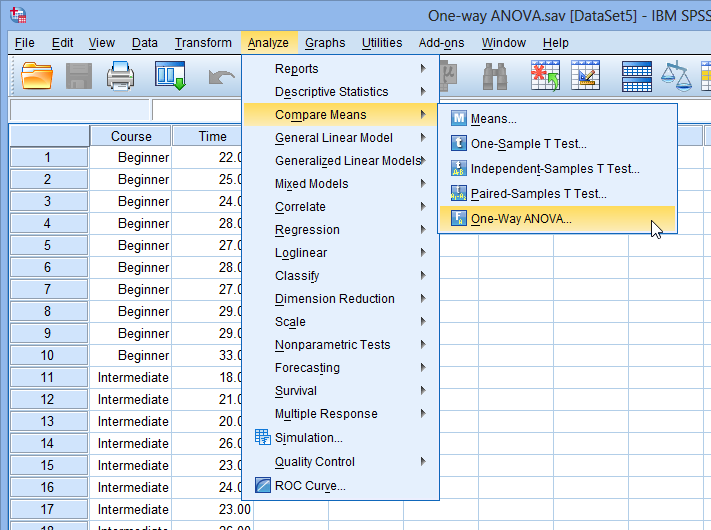Published with written permission from SPSS Statistics, IBM Corporation.

• You will be presented with the One-Way ANOVA dialogue box: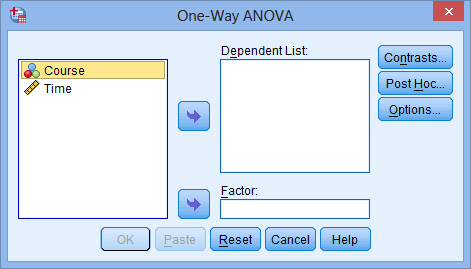Published with written permission from SPSS Statistics, IBM Corporation.

• Transfer the dependent variable, Time, into the Dependent List: box and the independent variable, Course, into the Factor: box using the appropriate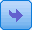buttons (or drag-and-drop the variables into the boxes), as shown below: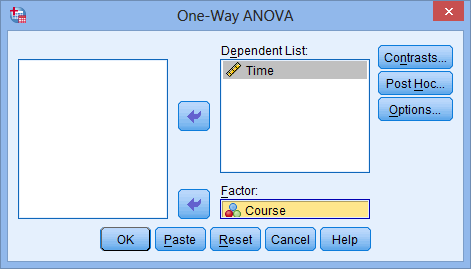Published with written permission from SPSS Statistics, IBM Corporation.

• Click the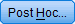button. Tick the Tukey checkbox as shown below: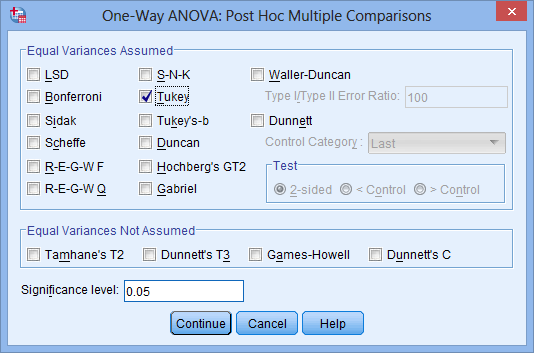Published with written permission from SPSS Statistics, IBM Corporation.

• Click the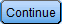button.

# Access all 95 SPSS Statistics guides in Laerd Statistics• Click the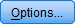button. Tick the Descriptive checkbox in the –Statistics– area, as shown below: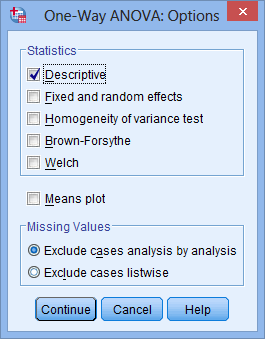Published with written permission from SPSS Statistics, IBM Corporation.

NOTE: When testing for some of the assumptions of the one-way ANOVA, you will need to tick more of these checkboxes. We take you through this, including how to interpret the output, in our enhanced one-way ANOVA guide.

• Click thebutton.

• Click the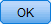button.

Go to the next page for the SPSS Statistics output and an explanation of the output.